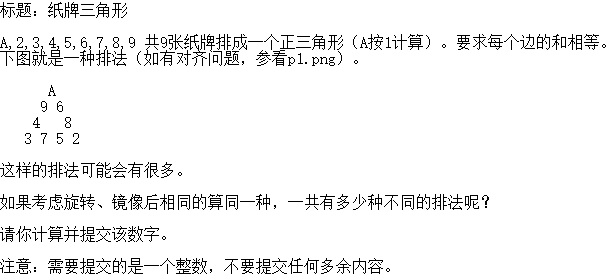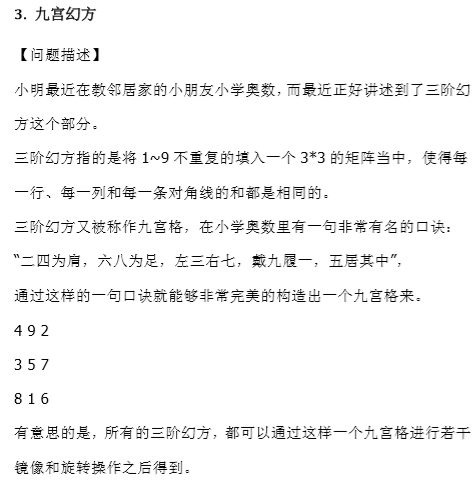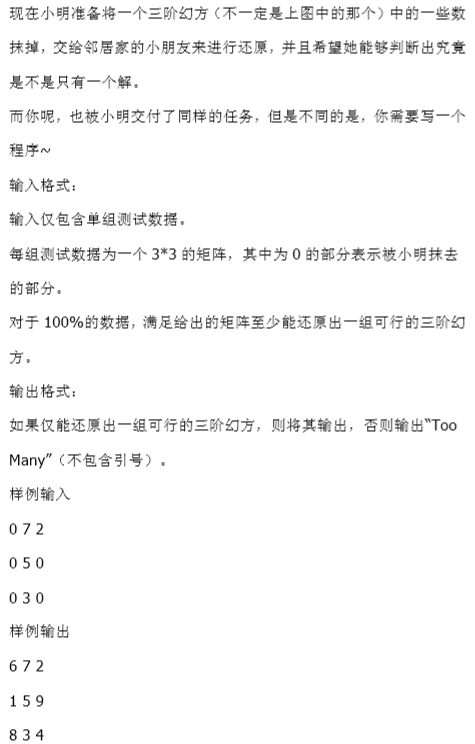# 纸牌三角形and九宫幻方一些对称数组图形关于旋转和镜像的一些规律总结

①纸牌三角形
②九宫幻方import java.util.Arrays;

public class Two {
static int cnt = 0;
public static void main(String[] args) {
do {
int sum1 = Arrays + Arrays + Arrays + Arrays;
int sum2 = Arrays + Arrays + Arrays + Arrays;
int sum3 = Arrays + Arrays + Arrays + Arrays;
if (sum1==sum2&&sum2==sum3) {
cnt++;
}
} while (next_permutation(Arrays));
System.out.println(cnt/6);
}
// 方法一 使用 next_permutation
public static void swap(int nums[], int left, int right) {
int t = nums[left];
nums[left] = nums[right];
nums[right] = t;
}
public static void reverse(int nums[], int left, int right) {

while (left < right) {
swap(nums, left++, right--);
}
}
public static boolean next_permutation(int nums[]) {
for (int i = nums.length - 2; i >= 0; i--) {
if (nums[i] < nums[i + 1]) {
for (int j = nums.length - 1; j > i; j--) {
if (nums[j] > nums[i]) {
swap(nums, i, j);
reverse(nums, i + 1, nums.length - 1);
return true;
}
}
}
}
return false;
}
static int Arrays[] = { 1, 2, 3, 4, 5, 6, 7, 8, 9 };
}04-161万+
02-14115209-23765
03-31462
04-14263
05-11870
02-22206
07-291万+
08-1690
05-125502
04-10393
03-081963
03-28360
03-04177
11-181万+
03-0366
03-131347
©️2020 CSDN 皮肤主题: 大白 设计师:blogdevteam